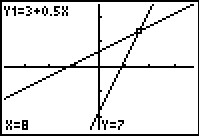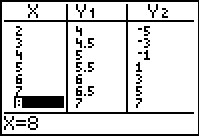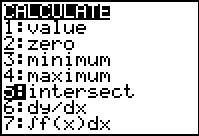# Activities

••• ##### Subject Area

• Math: Algebra I: Systems of Linear Equations

• ##### Author9-12

30 Minutes

• ##### Device
• TI-83 Plus Family
• TI-84 Plus
• TI-84 Plus Silver Edition
•TI-84 Plus C Silver Edition
•TI-84 Plus CE
• ##### Report an Issue

Understanding Solutions of Systems Using Tables and Graphs#### Activity Overview

Students represent and analyze mathematical situations and structures using algebraic symbols.

#### Key Steps

•Students will understand how the solution of a system of two equations is represented graphically and in a table of values. They will first use the trace feature to estimate the intersection of the two equations.

•Then students will set up the table and use it to find the x-value that produces the same y-value for both Y1 and Y2.

•The intersect command will enable students to verify the coordinates of the solution they found using the table of values.